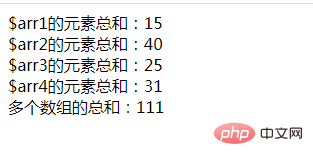# php中多个数组怎么求和array_sum()是PHP中的一个内置函数，可以计算数组中所有元素的和，并返回元素之和。

```\$sum1=array_sum(\$arr1);
\$sum2=array_sum(\$arr2);
\$sum3=array_sum(\$arr3);
\$sum4=array_sum(\$arr4);
...
\$sumN=array_sum(\$arrN);```

`\$sum = \$sum1 + \$sum2 + \$sum3 + \$sum4 +....+ \$sumN;`

```<?php
\$arr1 = array(1,2,3,4,5);
\$arr2 = array(6,7,8,9,10);
\$arr3 = array(1,3,5,7,9);
\$arr4 = array(2,4,6,9,10);

\$sum1=array_sum(\$arr1);
echo '\$arr1的元素总和：'.\$sum1;
\$sum2=array_sum(\$arr2);
echo '<br>\$arr2的元素总和：'.\$sum2;
\$sum3=array_sum(\$arr3);
echo '<br>\$arr3的元素总和：'.\$sum3;
\$sum4=array_sum(\$arr4);
echo '<br>\$arr4的元素总和：'.\$sum4;

\$sum = \$sum1 + \$sum2 + \$sum3 + \$sum4;

echo '<br>多个数组的总和：'.\$sum;
?>```1/1